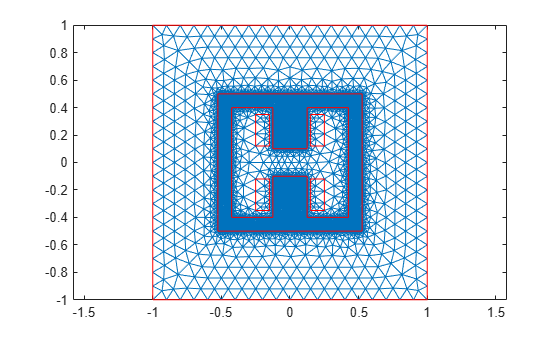Magnetic Flux Density in H-Shaped Magnet

This example shows how to solve a 2-D magnetostatic model for a ferromagnetic frame with an H-shaped cavity. This setup generates a uniform magnetic field due to the presence of two coils.

Create a geometry that consists of a rectangular frame with an H-shaped cavity, four rectangles representing the two coils, and a unit square representing the air domain around the magnet. Specify all dimensions in millimeters, and use the value convfactor = 1000 to convert the dimensions to meters.

convfactor = 1000;

Create the H-shaped geometry to model the cavity.

xCoordsCavity = [-425 -125 -125 125 125 425 425 ...
125 125 -125 -125 -425]/convfactor;
yCoordsCavity = [-400 -400 -100 -100 -400 -400 ...
400 400 100 100 400 400]/convfactor;
RH = [2;12;xCoordsCavity';yCoordsCavity'];

Create the geometry to model the rectangular ferromagnetic frame.

RS = [3;4;[-525;525;525;-525;-500;-500;500;500]/convfactor];

Create the geometries to model the coils.

RC1 = [3;4;[150;250;250;150;120;120;350;350]/convfactor;
RC2 = [3;4;[-150;-250;-250;-150;120;120;350;350]/convfactor;
RC3 = [3;4;[150;250;250;150;-120;-120;-350;-350]/convfactor;
RC4 = [3;4;[-150;-250;-250;-150;-120;-120;-350;-350]/convfactor;

Create the geometry to model the air domain around the magnet.

RD = [3;4;[-1000;1000;1000;-1000;-1000; ...

Combine the shapes into one matrix.

gd = [RS,RH,RC1,RC2,RC3,RC4,RD];

Create a set formula and create the geometry.

ns = char('RS','RH','RC1','RC2','RC3','RC4','RD');
g = decsg(gd,'(RS+RH+RC1+RC2+RC3+RC4)+RD',ns');

Plot the geometry with the face labels.

figure
pdegplot(g,'FaceLabels','on')Plot the geometry with the edge labels.

figure
pdegplot(g,'EdgeLabels','on')Create a magnetostatic model and include the geometry in the model.

model = createpde('electromagnetic','magnetostatic');
geometryFromEdges(model,g);

Generate a mesh with fine refinement in the ferromagnetic frame.

figure
pdemesh(model)Specify the vacuum permeability value in the SI system of units.

model.VacuumPermeability = 1.2566370614E-6;

Specify a relative permeability of 1 for all domains.

electromagneticProperties(model,'RelativePermeability',1);

Now specify the large relative permeability of the ferromagnetic frame.

electromagneticProperties(model,'RelativePermeability',10000,'Face',2);

Specify the current density values on the upper and lower coils.

electromagneticSource(model,'CurrentDensity',1E6,'Face',[5,6]);
electromagneticSource(model,'CurrentDensity',-1E6,'Face',[4,7]);

Specify that the magnetic potential on the outer surface of the air domain is 0.

electromagneticBC(model,'Edge',[5,6,13,14],'MagneticPotential',0);

Solve the model.

R = solve(model)
R =
MagnetostaticResults with properties:

MagneticPotential: [26381x1 double]
MagneticField: [1x1 FEStruct]
MagneticFluxDensity: [1x1 FEStruct]
Mesh: [1x1 FEMesh]

Plot the magnitude of the flux density.

Bmag = sqrt(R.MagneticFluxDensity.Bx.^2 + ...
R.MagneticFluxDensity.By.^2);

pdeplot(model,'XYData',Bmag, ...
'FlowData',[R.MagneticFluxDensity.Bx ...
R.MagneticFluxDensity.By])References

 Kozlowski, A., R. Rygal, and S. Zurek. "Large DC electromagnet for semi-industrial thermomagnetic processing of nanocrystalline ribbon." IEEE Transactions on Magnetics 50, issue 4 (April 2014): 1-4. https://ieeexplore.ieee.org/document/6798057.

Partial Differential Equation Toolbox DocumentationGet trial now